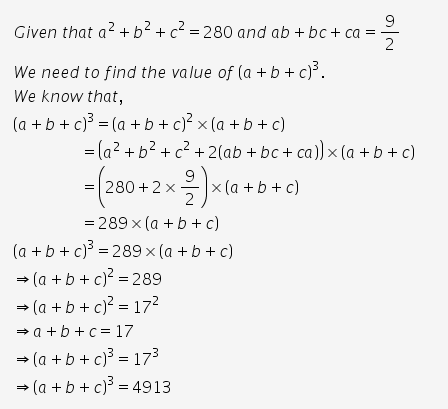# If a2 + b2 +c2 =280 and ab+bc+ca = 9 /2 find the value of ( a +b +c)3

if a2 + b2 +c2 =280 and ab+bc+ca = 9 /2 find the value of ( a +b +c)3